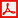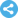Computational and Applied Mathematics Journal
Manuscript Information

Partial Chain Topologies on Finite Sets
Computational and Applied Mathematics Journal
Vol.1 , No. 4, Publication Date: Jun. 29, 2015, Page: 174-179
2571 Views Since June 29, 2015, 1232 Downloads Since Jun. 29, 2015Paper in PDF (311K)Follow on

Authors

  G. A. Kamel, Department of Mathematics, Faculty of Science, Fayoum University, Fayoum, Egypt.

Abstract

Although, there are lots of equivalent ways of formulating for computing the number of topological spaces in finite set. In this paper, we formulated special case for computing the number of chain topological spaces, and maximal elements with the natural generalization. We look at the concept of partial chain topologies on finite set with respect to the given subset. We determine the number of partial chain topologies with k open sets, and so the number of all chain topologies on finite set will be outlined. To support our study, some examples, and properties of this concept will be studied. Moreover, we determine the rule for computing the number of all maximal elements in the set of all chain topologies.

Keywords

Chain Topology, Partial Chain Topology, Number of Chain Topologies on Finite Set, Maximal Elements in Chain Topologies

Reference

  Benjamin, A. & Quinn, J. (2003). Proofs that really count. Washington, DC: Mathematical Association of America.  Brown J. I. and Watson S., The number of complements of a topology on n points is at least 2^n (except for some special cases), Discr. Math., 154 (1996), 27-39.  Clark, T. & Richmond, T. (2010). Collections of mutually disjoint convex subsets of a totally ordered set. Fibonacci Quarterly, 48, 77-79.  Ern´e, M. &Stege, K. (1991). Counting finite posets and topologies. Order, 8(3), 247-265.  Hwang, F. K. & Mallows, C. L. (1995). Enumerating nested and consecutive partitions. Journal of Combinatorial Theory, Series A 70, 323-333.  Kleitman D. J. and Rothschild B. L., The number of finite topologies, Proc. Amer. Math. Soc., 25 (1970), 276-282.  Kolli M., On the cardinality of the T0-topologies on a finite set, Preprint, 2014.  Messaoud Kolli, "Direct and Elementary Approach to Enumerate Topologies on a Finite Set", J. Integer Sequences, Volume 10, 2007, Article 07.3.1.  Moussa Benoumhani, The Number of Topologies on a Finite Set, Journal of Integer Sequences, Vol. 9 (2006), Article 06.2.6.  Richmond, B. (2000). Semigroups and their topologies arising from Green’s left quasiorder. Applied General Topology, 9 (2), 143-168.  Richmond T. (1998). Quasiorders, principal topolgies, and partially ordered partitions. International Joural of Mathematics and Mathematical Science 21 (2), 221-234.  Shafaat A., On the number of topologies definable for a finite set, J. Austral. Math. Soc., 8 (1968), 194-198.  Sharp H., Jr., Cardinality of finite topologies, J. Combinatorial Theory 5 (1968), 82-86.  Sloane, N. J. A. (2010). A007052: Number of order-consecutive partitions of n. The online encyclopedia of integer sequences. Retrieved (2010, March 30) from http://www.research.att.com/~njas/sequences/index.html.  Sloane, N. J. A. (2010). A000798: Number of different quasi-orders (or topologies, or transitive digraphs) with n labeled elements.. The On-line encyclopedia of integer sequences. Retrieved (2010, March 30) from http://www.research.att.com/ njas/sequences/A000798.  Stanley R., On the number of open sets of finite topologies, J. Combinatorial Theory 10 (1971) 75-79.  Stephen D., Topology on finite sets, Amer. Math. Monthly 75 (1968) 739-741.